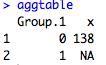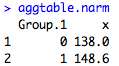## Monday, October 8, 2012

### Summarizing Data

In this post, I'll go over four functions that you can use to nicely summarize your data.  Before any regression analysis, a descriptive analysis is key to understanding your variables and the relationships between them.  Next week, I'll have a post on plotting, so this post is limited to the summary(), table(), and aggregate() functions.

Here is my dataset for this example:

The first thing I want to do is look at my data overall - get the range of values for each variable, and see what missing values I have.  I can do this simply by doing:

summary(mydata)

This produces the output below, and shows me that both Weight and Height have missing values. The Migrantstatus variable is a factor (categorical), so it lists the number in each category.If I want to just summarize one variable, I can do summary(mydata\$Weight) for example. And remember from last week, that if I just want to summarize some portion of my data, I can subset using indexing like so:  summary(mydata[,c(2:5)])

Next, I want to tabulate my data.  I can do univariate and bivariate tables (I can even do more dimensions than that!) by using the table() function.  Table() gives me the totals in each group.  If I want proportions instead of totals, I can use prop.table() around my table() function.  The code looks like this with the output below:

table1<-table(mydata\$Sex)

table1
prop.table(table1)Next, I do the bivariate tables.  The first variable is the row and the second is the column.  I can do proportions here as well, but I must be careful about the margin.  Margin=1 means that R calculates the proportions across rows, while margin=2 is down columns. I show a table of Sex vs Marital status below with two types of proportion tables.

table2<-table(mydata\$Sex, mydata\$Married)

table2
prop.table(table2, margin=1)
prop.table(table2, margin=2)

And if I want to do three dimensions, I put all three variables in my table() function.  R will give me the 2x2 table of sex and marital status, stratified by the third variable, migrant status.

table3<-table(mydata\$Sex, mydata\$Married, mydata\$Migrantstatus)

The great part about R is that I can take any component of this table that I want.  For example, if I just want the table for migrants, I can do:

table3[,,1]

which tells R to give me all rows and columns, but only for the first category of the third variable.  I get the following output, which you can see is the same as the first part of table 3 from above.

Finally, what if I want to calculate the mean of one variable by another variable? One way to do this is to use the aggregate() function.  Aggregate does exactly that: it takes one variable (the first argument) and calculates some kind of function on it (the FUN= argument), by another variable (the by=list() argument).  So here I am going to do the mean weight for each sex.  Here the syntax is a little funny because R wants a list for the by variable.  I will go over lists at another post in the future, or you can look it up on another R site.

aggtable<-aggregate(mydata\$Weight, by=list(mydata\$Sex), FUN=mean)
aggtableHowever, something is wrong. The NA in the weight column is messing up my mean calculation.  To get around this, I use the na.rm=TRUE parameter which removes any NAs from consideration.

aggtable.narm<-aggregate(mydata\$Weight, by=list(mydata\$Sex), FUN=mean, na.rm=TRUE)
aggtable.narmVictory! If I want to name my columns of this table, I can do:

names(aggtable.narm)<-c("Sex","Meanweight")

And of course if you want to do mean tables by more than one variable, you can put the all in the list argument, like so: by=list(mydata\$Sex, mydata\$Married).  The code and output would look like this:

aggtable.3<-aggregate(mydata\$Weight, by=list(mydata\$Sex, mydata\$Married), FUN=mean, na.rm=TRUE)

names(aggtable.3)<-c("Sex","Married","Meanweight")

aggtable.31.2.3.